# Class 8 NCERT Solutions – Chapter 3 Understanding Quadrilaterals – Exercise 3.1

• Last Updated : 11 Dec, 2020

### Question 1. Given here are some figures: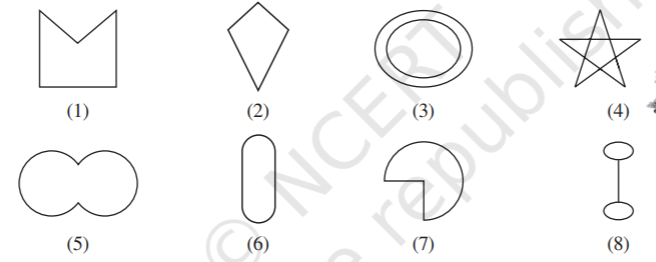a) 1, 2, 5, 6, 7

b) 1, 2, 5, 6, 7

c) 1, 2

d) 2

e) 1

### Question 2. How many diagonals does each of the following have?

#### Solution: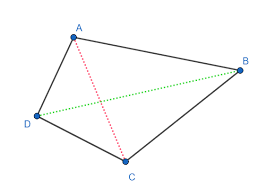Here we can see that only two diagonals are possible for the convex quadrilateral.

### (b) A regular hexagon

#### Solution: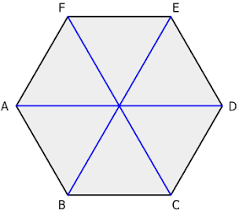Here we can see that only three diagonals are possible for the convex quadrilateral.

### (c) A triangle

#### Solution:In case of a triangle no diagonal is possible.

### Question 3. What is the sum of the measures of the angles of a convex quadrilateral? Will this property hold if the quadrilateral is not convex?

#### Solution:

Sum of the angles of a quadrilateral is always 180°. It doesn’t depend on whether the quadrilateral is a convex or a concave.

### Question 4. Examine the table. (Each figure is divided into triangles and the sum of the angles deduced from that).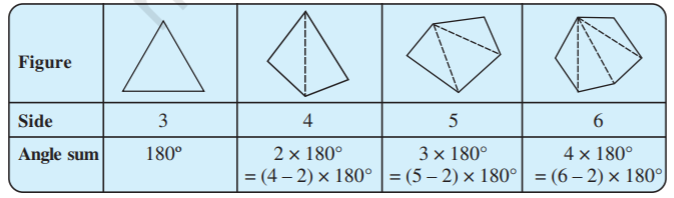### What can you say about the angle sum of a convex polygon with number of sides?

#### Solution:

From the above given table we can deduce that for a given number of sides n the sum of the angles of a convex polygon is (n – 2) × 180°.

a) 7

Ans: (7 – 2) × 180° = 5 × 180° = 900°

b) 8

Ans: (8 – 2) × 180° = 6 × 180° = 1080°

c) 10

Ans: (10 – 2) × 180° = 8 × 180° = 1440°

d) n

Ans: (n – 2) x 180°

### Question 5. What is a regular polygon? State the name of a regular polygon of : (i) 3 sides (ii) 4 sides (iii) 6 sides

#### Solution:

Regular Polygon is a polygon with equal sides and equal angles.

(i) 3 sides : Equilateral triangle

(ii) 4 sides : Square

(iii) 6 sides : Regular Hexagon

### Question 6. Find the angle measure x in the following figures.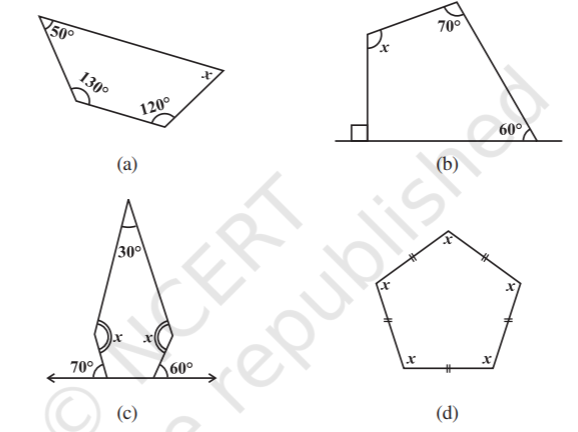#### Solution:

a) Total sum of the angles of the quadrilateral = 360°

Therefore, 50° + 130° + 120° + x = 360° ⇒ 300° + x = 360° ⇒ x = 60°

b) Total sum of the angles of the quadrilateral = 360°

Therefore, 60° + 70° + 90° + x = 360° ⇒ 220° + x = 360° ⇒ x = 140°

c) Total sum of the angles of the polygon = 540°

Angles adjacent to 60° = 180° – 60° = 120°

Angles adjacent to 70° = 180° – 70° = 110°

Therefore, 110° + 30° + 120° + x + x= 540° ⇒ 260° + 2x = 540° ⇒ 2x = 280° ⇒ x = 140°

d) Total sum of the angles of the polygon = 540°

Therefore, x° + x° + x° + x° + x°= 540° ⇒ 5x = 540° ⇒ x = 108°

### Question 7.  (a) Find x + y + z                                  (b) Find x + y + z + w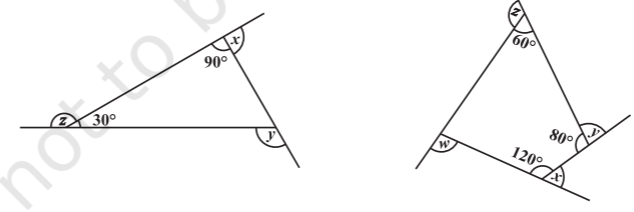#### Solution:

(a) By exterior angle property, y = 90° + 30° = 120°

x = 180° – 90° = 90°

z = 180° – 30° = 150°

So, the answer x + y + z = 360°

(b) Angle adjacent to w = 360° – ( 60° + 80° + 120° ) = 100°

w = 180° – 100°  = 80°

x = 180° – 120°  = 60°

y = 180° – 80° = 100°

z = 180° – 60° = 120°

So, the answer w + x + y + z = 360°

My Personal Notes arrow_drop_up